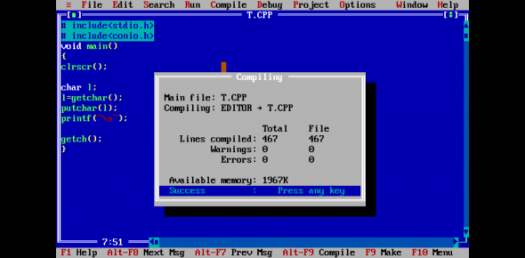# C Programming Quiz: Output Of C Programs! Trivia

10 Questions | Total Attempts: 123Settings“C” is a computer programming language, which supports structured programming, recursion, and lexical variable scope. It first appeared in 1972 and variations of it are still used prominently today. What do you know about it?

• 1.
)#include void call(int,int,int); int main(){  int a=10; call(a,a++,++a); return 0; } void call(int x,int y,int z){  printf("%d %d %d",x,y,z); }
• A.

10 10 12

• B.

12 11 11

• C.

12 12 12

• D.

10 11 12

• E.

Compiler error

• 2.
#include<stdio.h> int main(){ int a=-20; int b=-3; printf("%d",a%b); return 0; }
• A.

2

• B.

-2

• C.

18

• D.

-18

• E.

Compiler error

• 3.
#include<stdio.h> int main(){  char *url="c:\tc\bin\rw.c";  printf("%s",url); return 0; }
• A.

C:\tc\bin\rw.c

• B.

C:/tc/bin/rw.c

• C.

C: c inw.c

• D.

C:cinw.c

• E.

W.c in

• 4.
Which of the following is not derived data type in c?
• A.

Function

• B.

Pointer

• C.

Enumeration

• D.

Array

• E.

All the above

• 5.
The function which is used to move the file pointer to any desired location within a file?
• A.

Fseek()

• B.

Ftell()

• C.

Rewind()

• D.

Both a and b

• 6.
#include<stdio.h> int main(){  int a=15,b=10,c=5;  if(a>b>c )    printf("True");  else    printf("False"); return 0; }
• A.

True

• B.

False

• C.

Run time error

• D.

Compiler error

• E.

None of above

• 7.
What will be the output when you will execute following c code? #include<stdio.h> void main(){ char arr="Network"; printf("%s",arr); }
• A.

Network

• B.

N

• C.

Garbage value

• D.

Compilation error

• E.

None of the above

• 8.
#include<stdio.h> int main(){  const int i=5;  i++;  printf("%d",i); return 0; }
• A.

5

• B.

6

• C.

0

• D.

Compiler error

• E.

None of above

• 9.
#include<stdio.h> int main() { int i = 1; switch(i) { printf("Hello\n"); case 1: printf("Hi\n"); break; case 2: printf("\nBye\n"); break; } return 0; }
• A.

Hello hi

• B.

Hello bye

• C.

Hi

• D.

Hi hello

• E.

Hello

• 10.
# include<stdio.h> # define a 10 void foo(); main() {    printf("%d..",a);    foo();    printf("%d",a); } void foo() {    #undef a    #define a 50 }
• A.

10..10

• B.

10..50

• C.

Error

• D.

0

• E.

Compilation error

Related TopicsBack to top